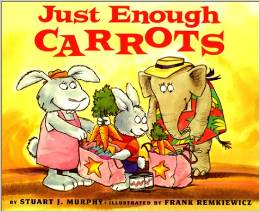# Less Than

5 teachers like this lesson
Print Lesson

## Objective

SWBAT model and compare two digit numbers to determine which is less.

#### Big Idea

Greator Gator, part 2! This lesson has students making models to compare two digit numbers.

## Activating Strategy

10 minutes

I like to start this lesson by reading the book Just Enough Carrots.Here is a You Tube video of the story.

I like to use this book because it really gets the kids involved in counting and understanding more and less than.  The graphics in this book also help students to understand the difference between same, fewer and more.

For the standard NBT.B.2.C, it is important for students to understand why a number is more or fewer than another number.  They need to have a firm understanding of the place value of the digits and be able to correctly compare numbers based on their place value.

On three index cards write 21, 15 and >.  Give the cards to three volunteers.  Have them arrange themselves in front of the class to make a greater than comparison.  Remind students that the pointed end points to the number that is less.  Discuss the comparison with the class.

• How do you read the comparison? (21 is greater than 15.)
• How do you know that 21 is greater? (21 has 2 tens and 15 has 1 ten.  So 21 is greater.)

If necessary, continue with different greater than comparisons and different volunteers.

## Teaching Strategies

15 minutes

Read the following problem to the class:

• Ron has 19 crackers.  Sam has 13 crackers.  Who has fewer crackers?  (Sam)  Which number is less, 19 or 13? (13)
• What is another number that is less than 19?  (accept any acceptable answer.)

Read the following problem to the class.  Have students use base ten blocks to model the two numbers:

• Which number is less, 22 or 28?
• How do you use your models to find which number is less? (Both have 2 tens, so I compare the ones.  22 has fewer ones than 28, so 22 is less than 28.)
• How is this like the process you used to find the number that is greater? (I compare the tens first, if the tens are the same, I compare the ones.)

Write the following on the board/chart paper:

43    <     49

﻿Have students draw a model to show how to represent the tens and ones.  Discuss how the tens are the same, so we need to compare the ones.

Discuss:

• Which number does the is less than symbol point to? (It points to 43, the number that is less.)

Have students read aloud the comparison, “43 is less than 49”

• How is the less than symbol different than the is greater than symbol? (It points in the opposite direction, it points to the left.)

## Independent Practice

30 minutes

For the independent practice portion of this lesson, I like to have the students complete a worksheet.

For struggling students, allow them to use base ten blocks to help with modeling the numbers that they need to compare.

## Closing/Summarizing

5 minutes

To close out this lesson, I make index cards with random numbers from 10-99 and give one to each student.  I then have students mix, pair, share and discuss which student has the lesser number, and why their number is less than the other number.